We are going to study Batch Norm, Weight Norm, Layer Norm, Instance Norm, Group Norm, Batch-Instance Norm, Switchable Norm{#591f}

Normalization has always been an active area of research in deep learning. Normalization techniques can decrease your model's training time by a huge factor. Let me state some of the benefits of using Normalization.{#5fbf}

1. It normalizes each feature so that they maintains the contribution of every feature, as some feature has higher numerical value than others. This way our network can be unbiased(to higher value features).{#5ea7} {#5ea7}
2. It reduces Internal Covariate Shift . It is the change in the distribution of network activations due to the change in network parameters during training. To improve the training, we seek to reduce the internal covariate shift.{#af62} {#af62}
3. In this paper, authors claims that Batch Norm makes loss surface smoother(i.e. it bounds the magnitude of the gradients much more tightly).{#ceb0} {#ceb0}
4. It makes the Optimization faster because normalization doesn't allow weights to explode all over the place and restricts them to a certain range.{#b5c7} {#b5c7}
5. An unintended benefit of Normalization is that it helps network in Regularization(only slightly, not significantly).{#02db} {#02db}

From above, we can conclude that getting Normalization right can be a crucial factor in getting your model to train effectively, but this isn't as easy as it sounds. Let me support this by certain questions.{#35dc}

1. How Normalization layers behave in Distributed training ?{#90a4} {#90a4}
2. Which Normalization technique should you use for your task like CNN, RNN, style transfer etc ?{#c737} {#c737}
3. What happens when you change the batch size of dataset in your training ?{#81be} {#81be}
4. Which norm technique would be the best trade-off for computation and accuracy for your network ?{#6c84} {#6c84}

To answer these questions, Let's dive into details of each normalization technique one by one.{#b506}

Batch normalization is a method that normalizes activations in a network across the mini-batch of definite size. For each feature, batch normalization computes the mean and variance of that feature in the mini-batch. It then subtracts the mean and divides the feature by its mini-batch standard deviation.{#3107}

But wait, what if increasing the magnitude of the weights made the network perform better?{#b01d}

To solve this issue, we can add γ and β as scale and shift learn-able parameters respectively. This all can be summarized as:{#436e}

ε是等式中的稳定常数。

Problems associated with Batch Normalization : {#e257}

1. Variable Batch Size → If batch size is of 1, then variance would be 0 which doesn't allow batch norm to work. Furthermore, if we have small mini-batch size then it becomes too noisy and training might affect. There would also be a problem in distributed training . As, if you are computing in different machines then you have to take same batch size because otherwise γ and β will be different for different systems.{#2096} {#2096}
2. Recurrent Neural Network → In an RNN, the recurrent activations of each time-step will have a different story to tell(i.e. statistics). This means that we have to fit a separate batch norm layer for each time-step. This makes the model more complicated and space consuming because it forces us to store the statistics for each time-step during training.{#4782} {#4782}

Batch norm alternatives(or better

s）将在下面详细讨论，但如果您只对非常简短的描述感兴趣（或仅通过查看图像进行修订），请查看：{＃1d8e}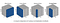This image is taken from https://arxiv.org/pdf/1803.08494.pdf

Wait, why don't we
Wait, why don't we normalize weights of a layer instead of normalizing the activations directly. Well,

Weight normalization reparameterizes the weights (

) as :{#92cf}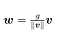It separates the weight vector from its direction, this has a similar effect as in batch normalization with variance. The only difference is in variation instead of direction.{#b12c}

As for the mean, authors of this paper cleverly combine

and weight normalization to get the desired output even in small mini-batches. It means that they subtract out the mean of the minibatch but do not divide by the variance. Finally, they use weight normalization instead of dividing by variance.{#3b01}

Note: Mean is less noisy as compared to variance(which above makes mean a good choice over variance) due to the

. {#ea19}
{#ea19}

The paper shows that weight normalization combined with mean-only batch normalization achieves the best results on CIFAR-10.{#b097}

Layer normalization normalizes input across the features instead of normalizing input features across the batch dimension in batch normalization.{#f031}

A mini-batch consists of multiple examples with the same number of features. Mini-batches are matrices(or tensors) where one axis corresponds to the batch and the other axis(or axes) correspond to the feature dimensions.{#5d92}

The authors of the paper claims that layer normalization performs better than batch norm in case of

s.{#acbb}

Layer normalization and

Here,
Here, x ∈ ℝ T ×C×W×H be an input tensor containing a batch of T images. Let
∈ℝT×C×W×H是包含一批T图像的输入张量。让 x ₜᵢⱼₖ denote its tijk-th element, where k and j span spatial dimensions(
ₜᵢⱼₖ表示其tijk-th元素，其中k和j跨越空间维度（ H eight and
eight and W idth of the image), i is the feature channel (color channel if the input is an RGB image), and t is the index of the image in the batch.{#ec58}

This technique is originally devised for

，问题实例规范化试图解决的问题是网络应该是不可知的 contrast of the original image.{#66d3}

As the name suggests,

∵ When we put all the channels into a single group, group normalization becomes Layer normalization. And, when we put each channel into different groups it becomes Instance normalization. {#f54e}
{#f54e}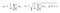Sᵢ is defined below.
Sᵢ定义如下。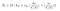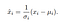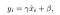Here,
Here, x is the feature computed by a layer, and i is an index. In the case of 2D images,

= ( i N ,
N , i C ,
C , i H,
H, i W ) is a 4D vector indexing the features in (N, C, H, W) order, where N is the batch axis,
W）是以（N，C，H，W）顺序索引特征的4D向量，其中N是批轴， C is the channel axis, and

and W are the spatial height and width axes.

"指的是指数 i and
and k are in the same group of channels, assuming each group of channels are stored in a sequential order along the C axis. GN computes µ and σ along the (H, W) axes and along a group of C/G channels.{#a539}

The problem with Instance normalization is that it completely erases style information. Though, this has its own merits(such as in style transfer) it can be problematic in those conditions where contrast matters(like in weather classification, brightness of the sky matters). Batch-instance normalization attempts to deal with this by learning how much style information should be used for each channel(

).{#750c}

Batch-Instance Normalization is just an interpolation between batch norm and instance norm.{#0820}

ρ的值在0和1之间。

The interesting aspect of batch-instance normalization is that the balancing parameter ρ is learned through gradient descent.{#0eb0}

From batch-instance normalization, we can conclude that models could learn to adaptively use different normalization methods using gradient descent. {#37fa}
{#37fa}

Understanding from above, a question may arise.{#a707}

Can we switch the normalization technique whenever needed ? {#9c1b}

This paper proposed switchable normalization, a method that uses a weighted average of different mean and variance statistics from batch normalization, instance normalization, and layer normalization.{#72c4}

The authors showed that switch normalization could potentially outperform batch normalization on tasks such as image classification and object detection.{#ec51}

The paper showed that the instance normalization were used more often in earlier layers, batch normalization was preferred in the middle and layer normalization being used in the last more often. Smaller batch sizes lead to a preference towards layer normalization and instance normalization.{#de24}

(未经同意，请勿转载)
(未经同意，请勿转载)